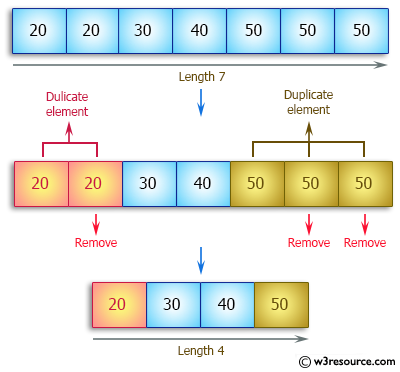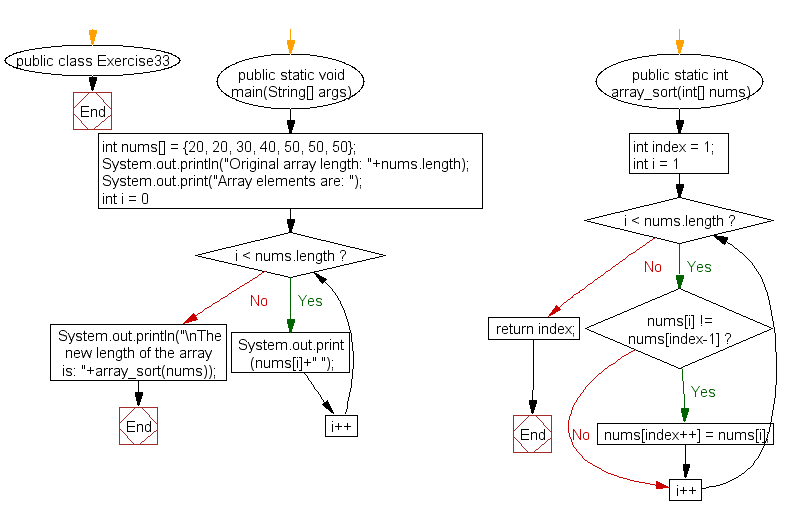﻿ Java: Remove duplicate elements from a given array# Java Array Exercises: Remove the duplicate elements of a given array and return the new length of the array

## Java Array: Exercise-33 with Solution

Write a Java program to remove the duplicate elements of a given array and return the new length of the array.
Sample array: [20, 20, 30, 40, 50, 50, 50]
After removing the duplicate elements the program should return 4 as the new length of the array.

Pictorial Presentation:Sample Solution:

Java Code:

``````public class Exercise33 {
public static void main(String[] args) {
int nums[] = {20, 20, 30, 40, 50, 50, 50};
System.out.println("Original array length: "+nums.length);
System.out.print("Array elements are: ");
for (int i = 0; i < nums.length; i++)
{
System.out.print(nums[i]+" ");
}
System.out.println("\nThe new length of the array is: "+array_sort(nums));

}

public static int array_sort(int[] nums) {
int index = 1;
for (int i = 1; i < nums.length; i++) {
if (nums[i] != nums[index-1])
nums[index++] = nums[i];
}
return index;
}
}
```
```

Sample Output:

```
Original array length: 7
Array elements are: 20 20 30 40 50 50 50
The new length of the array is: 4
```

Flowchart:Visualize Java code execution (Python Tutor):

Java Code Editor:

Improve this sample solution and post your code through Disqus

What is the difficulty level of this exercise?

Test your Programming skills with w3resource's quiz.

﻿

## Java: Tips of the Day

countOccurrences

Counts the occurrences of a value in an array.

Use Arrays.stream().filter().count() to count total number of values that equals the specified value.

```public static long countOccurrences(int[] numbers, int value) {
return Arrays.stream(numbers)
.filter(number -> number == value)
.count();
}
```

Ref: https://bit.ly/3kCAgLb